Here Chapter 8 Quadrilaterals Class 9 Maths NCERT Solutions are available that will be helpful in understanding the key concepts in an easy way. Here you can download PDF of NCERT Solutions for Class 9 Maths Chapter 8 Quadrilaterals that will serve as beneficial tool that can be used to recall various questions any time. You can also complete your homework on time through the help of these Chapter 8 NCERT Solutions and able to solve the difficult problems in a given in a exercise.

For application of concepts, an individual should first need to focus on the Quadrilaterals NCERT Solutions as it will tell you about the difficulty of questions. These NCERT Solutions will help an individual to increase concentration and you can solve questions of supplementary books easily.Page No: 146

Exercise 8.1

1. The angles of quadrilateral are in the ratio 3 : 5 : 9 : 13. Find all the angles of the quadrilateral.

Let x be the common ratio between the angles.
Sum of the interior angles of the quadrilateral = 360°
Now,
3x + 5x + 9x + 13x = 360°
⇒ 30x = 360°
⇒ x = 12°
3x = 3×12° = 36°
5x = 5×12° = 60°
9x = 9×12° = 108°
13x = 13×12° = 156°

2. If the diagonals of a parallelogram are equal, then show that it is a rectangle.Given,
AC = BD
To show,
To show ABCD is a rectangle we have to prove that one of its interior angle is right angled.
Proof,
BC = BA (Common)
AC = AD (Opposite sides of a parallelogram are equal)
AC = BD (Given)
Therefore, ΔABC ≅ ΔBAD by SSS congruence condition.
∠A = ∠B (by CPCT)
also,
∠A + ∠B = 180° (Sum of the angles on the same side of the transversal)
⇒ 2∠A = 180°
⇒ ∠A = 90° = ∠B
Thus ABCD is a rectangle.

3. Show that if the diagonals of a quadrilateral bisect each other at right angles, then it is a rhombus.Let ABCD be a quadrilateral whose diagonals bisect each other at right angles.
Given,
OA = OC, OB = OD and ∠AOB = ∠BOC = ∠OCD = ∠ODA = 90°
To show,
ABCD is parallelogram and AB = BC = CD = AD
Proof,
In ΔAOB and ΔCOB,
OA = OC (Given)
∠AOB = ∠COB (Opposite sides of a parallelogram are equal)
OB = OB (Common)
Therefore, ΔAOB ≅ ΔCOB by SAS congruence condition.
Thus, AB = BC (by CPCT)
Similarly we can prove,
AB = BC = CD = AD
Opposites sides of a quadrilateral are equal hence ABCD is a parallelogram.
Thus, ABCD is rhombus as it is a parallelogram whose diagonals intersect at right angle.

4. Show that the diagonals of a square are equal and bisect each other at right angles.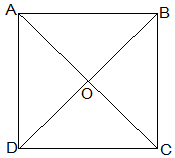Let ABCD be a square and its diagonals AC and BD intersect each other at O.
To show,
AC = BD, AO = OC and ∠AOB = 90°
Proof,
BC = BA (Common)
Therefore, ΔABC ≅ ΔBAD by SAS congruence condition.
Thus, AC = BD by CPCT. Therefore, diagonals are equal.
Now,
In ΔAOB and ΔCOD,
∠BAO = ∠DCO (Alternate interior angles)
∠AOB = ∠COD (Vertically opposite)
AB = CD (Given)
Therefore, ΔAOB ≅ ΔCOD by AAS congruence condition.
Thus, AO = CO by CPCT. (Diagonal bisect each other.)
Now,
In ΔAOB and ΔCOB,
OB = OB (Given)
AO = CO (diagonals are bisected)
AB = CB (Sides of the square)
Therefore, ΔAOB ≅ ΔCOB by SSS congruence condition.
also, ∠AOB = ∠COB
∠AOB + ∠COB = 180° (Linear pair)
Thus, ∠AOB = ∠COB = 90° (Diagonals bisect each other at right angles)

5. Show that if the diagonals of a quadrilateral are equal and bisect each other at right angles, then it is a square.Given,
Let ABCD be a quadrilateral in which diagonals AC and BD bisect each other at right angle at O.
To prove,
Proof,
In ΔAOB and ΔCOD,
AO = CO (Diagonals bisect each other)
∠AOB = ∠COD (Vertically opposite)
OB = OD (Diagonals bisect each other)
Therefore, ΔAOB ≅ ΔCOD by SAS congruence condition.
Thus, AB = CD by CPCT. --- (i)
also,
∠OAB = ∠OCD (Alternate interior angles)
⇒ AB || CD
Now,
In ΔAOD and ΔCOD,
AO = CO (Diagonals bisect each other)
∠AOD = ∠COD (Vertically opposite)
OD = OD (Common)
Therefore, ΔAOD ≅ ΔCOD by SAS congruence condition.
Thus, AD = CD by CPCT. --- (ii)
also,
⇒ AD = BC = CD = AB --- (ii)
also,  ∠ADC = ∠BCD  by CPCT.
and ∠ADC + ∠BCD = 180° (co-interior angles)
⇒ ∠ADC = 90° --- (iii)
One of the interior ang is right angle.
Thus, from (i), (ii) and (iii) given quadrilateral ABCD is a square.

6. Diagonal AC of a parallelogram ABCD bisects ∠A (see Fig. 8.19). Show that
(i) it bisects ∠C also,
(ii) ABCD is a rhombus.(i)
AD = CB (Opposite sides of a ||gm)
DC = BA (Opposite sides of a ||gm)
AC = CA (Common)
Therefore, ΔADC ≅ ΔCBA by SSS congruence condition.
Thus,
∠ACD = ∠CAB by CPCT
⇒ ∠ACD = ∠BCA
Thus, AC bisects ∠C also.

⇒ AD = CD (Opposite sides of equal angles of a triangle are equal)
Also, AB = BC = CD = DA (Opposite sides of a ||gm)
Thus, ABCD is a rhombus.

7. ABCD is a rhombus. Show that diagonal AC bisects ∠A as well as ∠C and diagonal BD bisects ∠B as well as ∠D.Let ABCD is a rhombus and AC and BD are its diagonals.
Proof,
AD = CD (Sides of a rhombus)
∠DAC = ∠DCA (Angles opposite of equal sides of a triangle are equal.)
also, AB || CD
⇒ ∠DAC = ∠BCA (Alternate interior angles)
⇒ ∠DCA = ∠BCA
Therefore, AC bisects ∠C.
Similarly, we can prove that diagonal AC bisects ∠A.

Also, by preceding above method we can prove that diagonal BD bisects ∠B as well as ∠D.

8. ABCD is a rectangle in which diagonal AC bisects ∠A as well as ∠C. Show that:
(i) ABCD is a square
(ii) diagonal BD bisects ∠B as well as ∠D.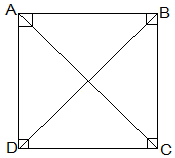(i)∠DAC = ∠DCA (AC bisects ∠A as well as ∠C)
⇒ AD = CD (Sides opposite to equal angles of a triangle are equal)
also, CD = AB (Opposite sides of a rectangle)
Therefore, AB = BC = CD = AD
Thus, ABCD is a square.

(ii) In ΔBCD,
BC = CD
⇒ ∠CDB = ∠CBD (Angles opposite to equal sides are equal)
also, ∠CDB = ∠ABD (Alternate interior angles)
⇒ ∠CBD = ∠ABD
Thus, BD bisects ∠B
Now,
Thus, BD bisects ∠D

Page No: 147

9. In parallelogram ABCD, two points P and Q are taken on diagonal BD such that DP = BQ (see Fig. 8.20). Show that:
(i) ΔAPD ≅ ΔCQB
(ii) AP = CQ
(iii) ΔAQB ≅ ΔCPD
(iv) AQ = CP
(v) APCQ is a parallelogram

(i) In ΔAPD and ΔCQB,
DP = BQ (Given)
∠ADP = ∠CBQ (Alternate interior angles)
AD = BC (Opposite sides of a ||gm)
Thus, ΔAPD ≅ ΔCQB by SAS congruence condition.

(ii) AP = CQ by CPCT as ΔAPD ≅ ΔCQB.

(iii) In ΔAQB and ΔCPD,
BQ = DP (Given)
∠ABQ = ∠CDP (Alternate interior angles)
AB = BCCD (Opposite sides of a ||gm)
Thus, ΔAQB ≅ ΔCPD by SAS congruence condition.

(iv) AQ = CP by CPCT as ΔAQB ≅ ΔCPD.

(v) From (ii)  and (iv), it is clear that APCQ has equal opposite sides also it has equal opposite angles. Thus, APCQ is a ||gm.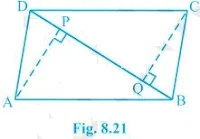10. ABCD is a parallelogram and AP and CQ are perpendiculars from vertices A and C on diagonal BD (see Fig. 8.21). Show that
(i) ΔAPB ≅ ΔCQD
(ii) AP = CQ

(i) In ΔAPB and ΔCQD,
∠ABP = ∠CDQ (Alternate interior angles)
∠APB = ∠CQD (equal to right angles as AP and CQ are perpendiculars)
AB = CD (ABCD is a parallelogram)
Thus, ΔAPB ≅ ΔCQD by AAS congruence condition.

(ii) AP = CQ by CPCT as ΔAPB ≅ ΔCQD.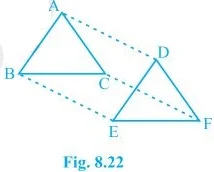11. In ΔABC and ΔDEF, AB = DE, AB || DE, BC = EF and BC || EF. Vertices A, B and C are joined to vertices D, E and F respectively (see Fig. 8.22).
Show that
(i) quadrilateral ABED is a parallelogram
(ii) quadrilateral BEFC is a parallelogram
(iv) quadrilateral ACFD is a parallelogram
(v) AC = DF
(vi) ΔABC ≅ ΔDEF.

(i) AB = DE and AB || DE (Given)
Thus, quadrilateral ABED is a parallelogram because two opposite sides of a quadrilateral are equal and parallel to each other.
(ii) Again BC = EF and BC || EF.
Thus, quadrilateral BEFC is a parallelogram.
(iii)  Since ABED and BEFC are parallelograms.
⇒ AD = BE and BE = CF (Opposite sides of a parallelogram are equal)
Also, AD || BE and BE || CF (Opposite sides of a parallelogram are parallel)

(iv) AD and CF are opposite sides of quadrilateral ACFD which are equal and parallel to each other. Thus, it is a parallelogram.

(v) AC || DF and AC = DF because ACFD is a parallelogram.
(vi) In ΔABC and ΔDEF,
AB = DE (Given)
BC = EF (Given)
AC = DF (Opposite sides of a parallelogram)
Thus, ΔABC ≅ ΔDEF by SSS congruence condition.

12. ABCD is a trapezium in which AB || CD and
AD = BC (see Fig. 8.23). Show that
(i) ∠A = ∠B
(ii) ∠C = ∠D
(iv) diagonal AC = diagonal BD
[Hint : Extend AB and draw a line through C parallel to DA intersecting AB produced at E.]

Construction: Draw a line through C parallel to DA intersecting AB produced at E.
(i) CE = AD (Opposite sides of a parallelogram)
Therefor, BC = CE
⇒ ∠CBE = ∠CEB
also,
∠A + ∠CBE = 180° (Angles on the same side of transversal and ∠CBE = ∠CEB)
∠B + ∠CBE = 180° (Linear pair)
⇒ ∠A = ∠B

(ii) ∠A + ∠D = ∠B + ∠C = 180° (Angles on the same side of transversal)
⇒ ∠A + ∠D = ∠A + ∠C (∠A = ∠B)
⇒ ∠D = ∠C

AB = AB (Common)
∠DBA = ∠CBA
Thus, ΔABC ≅ ΔBAD by SAS congruence condition.

(iv) Diagonal AC = diagonal BD by CPCT as ΔABC ≅ ΔBA.

Page No: 150

Exercise 8.2

1. ABCD is a quadrilateral in which P, Q, R and S are mid-points of the sides AB, BC, CD and DA (see Fig 8.29). AC is a diagonal. Show that :
(i) SR || AC and SR = 1/2 AC
(ii) PQ = SR
(iii) PQRS is a parallelogram.(i) In ΔDAC,
R is the mid point of DC and S is the mid point of DA.
Thus by mid point theorem, SR || AC and SR = 1/2 AC

(ii) In ΔBAC,
P is the mid point of AB and Q is the mid point of BC.
Thus by mid point theorem, PQ || AC and PQ = 1/2 AC
also, SR = 1/2 AC
Thus, PQ = SR

(iii) SR || AC - from (i)
and, PQ || AC - from (ii)
⇒ SR || PQ - from (i) and (ii)
also, PQ = SR
Thus, PQRS is a parallelogram.

2. ABCD is a rhombus and P, Q, R and S are the mid-points of the sides AB, BC, CD and DA respectively. Show that the quadrilateral PQRS is a rectangle.Given,
ABCD is a rhombus and P, Q, R and S are the mid-points of the sides AB, BC, CD and DA respectively.
To Prove,
PQRS is a rectangle.
Construction,
AC and BD are joined.
Proof,
In ΔDRS and ΔBPQ,
DS = BQ (Halves of the opposite sides of the rhombus)
∠SDR = ∠QBP (Opposite angles of the rhombus)
DR = BP (Halves of the opposite sides of the rhombus)
Thus, ΔDRS ≅ ΔBPQ by SAS congruence condition.
RS = PQ by CPCT --- (i)
In ΔQCR and ΔSAP,
RC = PA (Halves of the opposite sides of the rhombus)
∠RCQ = ∠PAS (Opposite angles of the rhombus)
CQ = AS (Halves of the opposite sides of the rhombus)
Thus, ΔQCR ≅ ΔSAP by SAS congruence condition.
RQ = SP by CPCT --- (ii)
Now,
In ΔCDB,
R and Q are the mid points of CD and BC respectively.
⇒ QR || BD
also,
P and S are the mid points of AD and AB respectively.
⇒ PS || BD
⇒ QR || PS
Thus, PQRS is a parallelogram.
also, ∠PQR = 90°
Now,
In PQRS,
RS = PQ and RQ = SP from (i) and (ii)
∠Q = 90°
Thus, PQRS is a rectangle.

3. ABCD is a rectangle and P, Q, R and S are mid-points of the sides AB, BC, CD and DA respectively. Show that the quadrilateral PQRS is a rhombus.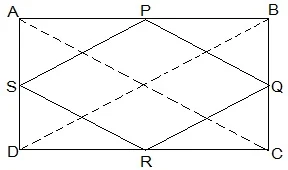Given,
ABCD is a rectangle and P, Q, R and S are mid-points of the sides AB, BC, CD and DA respectively.
Construction,
AC and BD are joined.
To Prove,
PQRS is a rhombus.
Proof,
In ΔABC
P and Q are the mid-points of AB and BC respectively
Thus, PQ || AC and PQ = 1/2 AC (Mid point theorem) --- (i)
SR || AC and SR = 1/2 AC (Mid point theorem) --- (ii)
So, PQ || SR and PQ = SR
As in quadrilateral PQRS one pair of opposite sides is equal and parallel to each other, so, it is a parallelogram.
PS || QR and PS = QR (Opposite sides of parallelogram) --- (iii)
Now,
In ΔBCD,
Q and R are mid points of side BC and CD respectively.
Thus, QR || BD and QR = 1/2 BD (Mid point theorem) --- (iv)
AC = BD (Diagonals of a rectangle are equal) --- (v)
From equations (i), (ii), (iii), (iv) and (v),
PQ = QR = SR = PS
So, PQRS is a rhombus.

4. ABCD is a trapezium in which AB || DC, BD is a diagonal and E is the mid-point of AD. A line is drawn through E parallel to AB intersecting BC at F (see Fig. 8.30). Show that F is the mid-point of BC.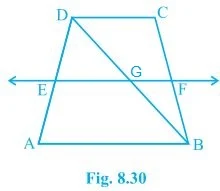Given,
ABCD is a trapezium in which AB || DC, BD is a diagonal and E is the mid-point of AD.
To prove,
F is the mid-point of BC.
Proof,
BD intersected EF at G.
E is the mid point of AD and also EG || AB.
Thus, G is the mid point of BD (Converse of mid point theorem)
Now,
In ΔBDC,
G is the mid point of BD and also GF || AB || DC.
Thus, F is the mid point of BC (Converse of mid point theorem)

Page No: 151

5. In a parallelogram ABCD, E and F are the mid-points of sides AB and CD respectively (see Fig. 8.31). Show that the line segments AF and EC trisect the diagonal BD.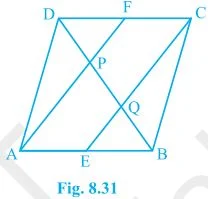Given,
ABCD is a parallelogram. E and F are the mid-points of sides AB and CD respectively.
To show,
AF and EC trisect the diagonal BD.
Proof,
ABCD is a parallelogram
Therefor, AB || CD
also, AE || FC
Now,
AB = CD (Opposite sides of parallelogram ABCD)
⇒ 1/2 AB = 1/2 CD
⇒ AE = FC (E and F are midpoints of side AB and CD)
AECF is a parallelogram (AE and CF are parallel and equal to each other)
AF || EC (Opposite sides of a parallelogram)
Now,
In ΔDQC,
F is mid point of side DC and FP || CQ (as AF || EC).
P is the mid-point of DQ (Converse of mid-point theorem)
⇒ DP = PQ --- (i)
Similarly,
In APB,
E is mid point of side AB and EQ || AP (as AF || EC).
Q is the mid-point of PB (Converse of mid-point theorem)
⇒ PQ = QB --- (ii)
From equations (i) and (i),
DP = PQ = BQ
Hence, the line segments AF and EC trisect the diagonal BD.

6. Show that the line segments joining the mid-points of the opposite sides of a quadrilateral bisect each other.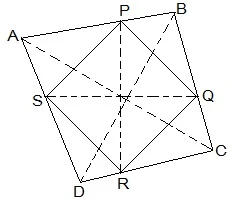Let ABCD be a quadrilateral and P, Q, R and S are the mid points of AB, BC, CD and DA respectively.
Now,
In ΔACD,
R and S are the mid points of CD and DA respectively.
Thus, SR || AC.
Similarly we can show that,
PQ || AC
PS || BD
QR || BD
Thus, PQRS is parallelogram.
PR and QS are the diagonals of the parallelogram PQRS. So, they will bisect each other.

7. ABC is a triangle right angled at C. A line through the mid-point M of hypotenuse AB and parallel to BC intersects AC at D. Show that
(i) D is the mid-point of AC

(ii) MD ⊥ AC
(iii) CM = MA = 1/2 AB(i) In ΔACB,
M is the mid point of AB and MD || BC
Thus, D is the mid point of AC (Converse of mid point theorem)

(ii) ∠ACB = ∠ADM (Corresponding angles)
also, ∠ACB = 90°
Thus, ∠ADM = 90° and MD ⊥ AC

(iii)  In ΔAMD and ΔCMD,
AD = CD (D is the midpoint of side AC)
DM = DM (common)
Thus, ΔAMD ≅ ΔCMD by SAS congruence condition.
AM = CM by CPCT
also, AM =  1/2 AB (M is mid point of AB)
Hence, CM = MA =  1/2 AB

## NCERT Solutions for Class 9 Maths Chapter 8 Quadrilaterals

Chapter 8 Quadrilaterals NCERT Solutions is very important for the preparation of exams. A figure formed by joining four points in an order is called a quadrilateral. A quadrilateral has four sides, four angles and four vertices. In this chapter, we will be discussing different types of quadrilaterals, their properties and about parallelograms.

• Angle Sum Property of a Quadrilateral: The sum of the angles of a quadrilateral is 360º. This can be verified by drawing a diagonal and dividing the quadrilateral into two triangles.

A square is a rectangle and also a rhombus.
A parallelogram is a trapezium.
A kite is not a parallelogram.
A trapezium is not a parallelogram (as only one pair of opposite sides is parallel in a trapezium and we require both pairs to be parallel in a parallelogram).
A rectangle or a rhombus is not a square.

• Properties of a Parallelogram:
1. A diagonal of a parallelogram divides it into two congruent triangles.
2. In a parallelogram, opposite sides are equal.
3. If each pair of opposite sides of a quadrilateral is equal, then it is a parallelogram.
4. In a parallelogram, opposite angles are equal.
5. If in a quadrilateral, each pair of opposite angles is equal, then it is a parallelogram.
6. The diagonals of a parallelogram bisect each other.
7. If the diagonals of a quadrilateral bisect each other, then it is a parallelogram.

• Another Condition for a Quadrilateral to be a Parallelogram: A quadrilateral is a parallelogram if a pair of opposite sides is equal and parallel.

• The Mid-point Theorem:
The line segment joining the mid-points of two sides of a triangle is parallel to the third side.
The line drawn through the mid-point of one side of a triangle, parallel to another side bisects the third side.

There are only two exercises in Chapter 8 Quadrilaterals NCERT Solutions which are provided below which can be helpful in completing your homework on time.

Studyrankers experts have taken every care while preparing these Class 9 Maths NCERT Solutions so you can easily clear your doubts. These NCERT Solutions are updated as per the latest marking scheme released by CBSE.

### NCERT Solutions for Class 9 Maths Chapters:

 Chapter 1 Number Systems Chapter 2 Polynomials Chapter 3 Coordinate Geometry Chapter 4 Linear Equations in Two Variables Chapter 5 Introduction to Euclid’s Geometry Chapter 6 Lines and Angles Chapter 7 Triangles Chapter 9 Areas of Parallelograms and Triangles Chapter 10 Circles Chapter 11 Constructions Chapter 12 Heron's Formula Chapter 13 Surface Areas And Volumes Chapter 14 Statistics Chapter 15 Probability

#### What are the benefits of NCERT Solutions for Chapter 8 Quadrilaterals Class 9 NCERT Solutions?

Through the help of NCERT Solutions of Chapter 8 Quadrilaterals you will be able to solve difficult questions easily and revising the chapter properly. It will improve the learning behaviour of the students and learning diverse topics.

#### What is a Rhombus?

A parallelogram having all sides equal is called a rhombus.

#### What is mid-point theorem?

The line segment joining the mid-points of any two sides of a triangle is parallel to the third side and equal to half of it.

#### In a quadrilateral, ∠ A : ∠ B : ∠ C : ∠ D = 1 : 2 : 3 : 4, then find the measure of each angle of the quadrilateral.

Since ∠ A : ∠ B : ∠ C : ∠ D = 1 : 2 : 3 : 4
∴ If ∠ A = x, then ∠ B = 2x, ∠ C = 3x and ∠ D = 4x. ∴ ∠ A + ∠ B + ∠ C + ∠ D = 360°
⇒ x + 2x + 3x + 4x = 360° ⇒ 10x = 36°
⇒ x= (360°/10)= 36°
∴ ∠ A = x = 36° ∠ B = 2x = 2 x 36° = 72° ∠ C = 3x = 3 x 36° = 108° ∠ D = 4x = 4 x 36° = 144°.